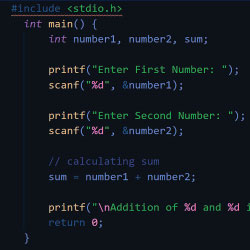# How to Generate Random Alphanumeric String in C#?

J

Janet

04 November 2021### Generate Random Alphanumeric String in C#

There are direct methods to Generate Random Numbers but there is no direct method to generate a random alphanumeric string, but if we want to generate a random alphanumeric string we can use some trick to implement this. Here in this answer, I'm going to introduce a simple trick to generate a random alphanumeric string, so let's see this.

#### Trick to Generate Random Alphanumeric String

In this trick, you need 2 things to generate an alphanumeric random string

•  An alphanumeric pre-defined string
• The length of random alphanumeric string that you want, Let's suppose we want 10 characters alphanumeric random string.

string base_string = "ffg45gt567dhgh6jkzas67a04nfd560424ggi5snw0bq9a184vxz2nr3";

int rs_lenght=10

string ranString = null; // final random alphanumeric string

Random rdn = new Random();

int rn_index;

int i;

for (i = 0; i < rs_lenght; i++)

{

rn_index = rdn.Next(base_string.Length);

ranString = ranString + base_string.ElementAt(rn_index);

}

From the above code, you will get the random alphanumeric string ( ranString )  which length will be 10 char, You can change the char in base_string and the length of output random alphanumeric string length by changing the value of rs_length.

I hope the above code will solve your problem, if you like my answer kindly vote for my answer.Top 10 most handsome Chinese actors
20 November 2021 | 12:00 AM